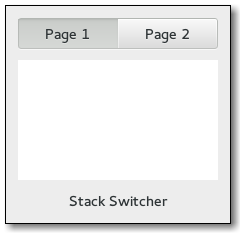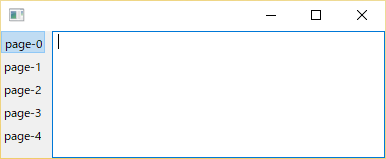# Stack window in wxPython

I’m looking for a window like this:What I’ve found are just notebooks, this is like a notebook that has buttons to switch between panels.

Hi, Patitotective

I think `wx.Toolbook` is close to your idea.

``````import wx

class Notebook(wx.Toolbook):
def __init__(self, parent):
super().__init__(parent, style=wx.BK_DEFAULT|wx.BK_LEFT)

self.li = wx.ImageList(1, 1) # dummy image list
bmp = wx.Bitmap(1,1)
self.AssignImageList(self.li)
for x in range(5):
win = wx.TextCtrl(self)
## imageId=wx.NO_IMAGE #=> wx._core.wxAssertionError: ..
imageId=0
)

app = wx.App()
frm = wx.Frame(None)
frm.nb = Notebook(frm)
frm.Show()
app.MainLoop()
``````Why do I need to asign an image list to the toolbox? I don’t understand that part.

``````import wx

class MyFrame(wx.Frame):
def __init__(self, **kwargs):
super().__init__(**kwargs)

toolbook = wx.Toolbook(self)
li = wx.ImageList(1, 1)
toolbook.AssignImageList(li)

for i in ("This is", "a toolbook"):
panel = wx.TextCtrl(toolbook, style=wx.TE_MULTILINE)

self.Show()

if __name__ == "__main__":
app = wx.App()
MyFrame(parent=None)
app.MainLoop()
``````

Also using the above example the pages are left aligned, I want them centered and using `toolboox.GetToolBar().AddStrechableSpace()` at the start and the end doesn’t seem to be working.

The one-pixel image is a dummy. From 4.1 and later, a zero-sized bitmap cannot be assigned or created (which will cause an assertion error), …unfortunately.For example, you can adjust the position such as

``````toolbook.ToolBar.Position = (100,0)
``````

after layout (i.e. `Show` in your code). You also need to bind wx.EVT_SIZE and calculate the position each time the window size is changed.

EDIT I like to write as `wx.Toolbook(self, style=wx.BK_TOP)`. (Explicit is better than implicit).